U.S. Department of Transportation
1200 New Jersey Avenue, SE
Washington, DC 20590
202-366-4000

Federal Highway Administration Research and Technology
Coordinating, Developing, and Delivering Highway Transportation InnovationsThis report is an archived publication and may contain dated technical, contact, and link information
 Federal Highway Administration > Publications > Research Publications > LTPP Publications > 02088 > 03.Cfm > Evaluation of Joint and Crack Load Transfer Final Report
 Publication Number: FHWA-RD-02-088 Date: May 2003

# Evaluation of Joint and Crack Load Transfer Final Report

This section presents the results of an assessment of FWD deflection data used in joint/crack LTE calculation and describes the procedure used to determine representative LTE parameters.

## Deflection Testing Details

The deflection data were downloaded during the summer of 2001 from LTPP database table MON_DEFL_DROP_DATA.  Information about sensor locations was obtained from database tables MON_DEFL_LOC_INFO and MON_DEFL_DEV_SENSORS (June 2001 release).  For rigid pavements in the LTPP program, the following types of deflection tests are conducted:

1. Center slab tests (J1 and C1 tests).
2. Corner tests (J2 and C2 tests).
3. Midpanel at the pavement edge tests (J3 and C3 tests).
4. LTE of joints/cracks tests (J4, J5, C4, and C5 tests).

For this study, only the LTE test data were used.  For the LTE testing, the FWD load is applied at one side of the joint or crack and the deflections are measured at both sides of the joint or crack.  The LTE testing with the load plate placed at the leave side of the joint requires deflection sensors placed at 0 and 305 mm (0 and 12 inches) from the center of the load plate.  The LTE testing with the load plate placed at the approach side of the joint requires deflection sensors placed at 0 and -305 mm (0 and -12 inches) from the center of the load plate.

The load sequence, as stored, for rigid pavement testing is as follows:

Drop Height No. of Drops Target Load, kN Acceptable Range, kN
2
3
4
4
4
4
40.0
53.3
71.1
36.0 to 44.0
48.1 to 58.7
64.1 to 78.3

For JCP, LTE tests are performed along the midlane path at each tested slab, and the test locations are designated as J4 for loads placed at the leave slab and J5 for loads placed at the approach slab.  The number of panels can vary from as few as 9 or 10 to as many as 35 or more on a 152.4-m (500-ft)-long section.  Regardless of the total number of panels present, no more than 20 panels are tested at one section.  For the CRCP, deflection basin tests are also performed along the midlane path at spacing of about 7.6 m (25 ft) and on both sides of a crack.  The test locations are designated as C4 and C5 for leave and approach panel loading, respectively.  For CRCP, two adjacent transverse cracks are typically at a spacing of 0.3 to 2.5 m (1 ft to 8 ft).  Tests are performed at 20 effective panels.

## Deflection Data Assessment

A total of 850,791 raw deflection basins were extracted from the LTPP database for 581 JCP sections and 116 CRCP sections, as shown in table 3.  The extracted data were examined to ensure their consistency and reasonableness, and some data were excluded from the analysis.  The reasons for data rejection were:

• Incorrect FWD sensor configuration.
• Incorrect testing time or location.
• Insufficient number of data points for a section.

Two percent of the basins (17,214 basins) were eliminated from the analysis.  LTEs were calculated for the remaining 833,577 basins, but 13,181 of them were identified as questionable and excluded from calculation of representative statistical indexes for joint and section LTEs.  The reason for the rejection was inconsistency with other measurements for the same time of testing, joint, load plate location, and load level.

The following procedure was used to examine the consistency of the FWD measurements.  First, for each time of testing, location, and FWD load and level, the average loaded and unloaded deflections and applied pressure were calculated.  After that, each FWD basin was tested on its deviation from the mean values.  The basin was rejected if at least one of the following conditions was violated: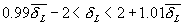(14)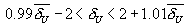(15)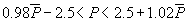(16)

where:

-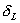= FWD sensor deflection on the loaded side of the crack/joint, microns.

-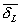= mean FWD sensor deflection on the loaded side of the crack/joint for the same time of testing, joint location, and FWD load level microns.

-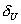= FWD sensor deflection on the loaded side of the crack/joint, microns.

-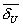= mean FWD sensor deflection on the loaded side of the crack/joint for the same FWD pass, joint location, and FWD load level, microns.

-          P and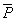= total FWD load and mean FWD load for the same FWD pass, joint location, and FWD load level in kN, respectively.  They are defined as follows: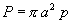(17)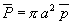(18)

where:

-          a = FWD plate radius, mm.

-          p and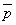= FWD pressure and mean FWD pressure for the same FWD pass, joint location, and FWD load level in kN, respectively.

Only 597 basins were rejected because of high variability in FWD load magnitude measurement.  Significantly more basins were rejected because of variability in loaded or unloaded deflection, as shown in table 2.

Table 2.  Number of basins rejected because of high variability.

Reason for Rejecting Number of Basins

High variability in FWD load magnitude

597

4,293

6,784

1,507

Overall, the quality of the deflection data was found to be very high.  More than 96 percent of the deflection basins measured for almost 700 sections were accepted for future analysis. Table 3 shows the distribution of the accepted and rejected basins for each test types.

Table 3.  Availability of deflection data.

Test Type Number of Sections Represented Number of Records Number of Excluded Records

C4 (approach)

116

69,025

847

C5 (leave)

116

65,172

805

J4 (approach)

581

355,825

7,243

J5 (leave)

572

343,555

4,286

Total

1,385

833,577

13,181

## Load Transfer Index Calculation Procedures

From the basins accepted after initial screening, representative LTE parameters were calculated for each deflection basin, for each joint, and for each FWD pass.  Analyses were performed separately for approach and leave tests.  Procedures for calculation of each set of parameters are presented below.

First, for each deflection basin extracted from the LTPP database, deflection LTE is calculated using equation 1.  After that, statistical summaries of LTE for each joint were computed.  This involved the following steps:

Step 1.  Compute mean LTE for joint/crack and FWD load level

For each FWD pass, joint/crack location, and drop height, mean LTE was computed.  Only LTEs from deflection basins that passed the criteria defined by equations (14) through (16) were used for computing these parameters.

Step 2.  Compute LTE crack/joint statistics

For each FWD pass and joint/crack location, the following parameters were computed:

• Mean joint/crack LTE.
• Minimum joint/crack LTE.
• Maximum joint/crack LTE.
• Standard deviation of joint/crack LTE.

LTEs from all FWD load levels were used to compute these parameters.

Step 3.  Determine joint/crack LTE load dependency index

Using mean LTE for nominal load levels of 40 kN (drop height equal to 2) and 70 kN (drop height equal to 4), LTE load dependency index was identified using the following criteria: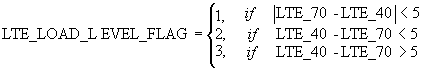(19)

where:

-          LTE_LOAD_LEVEL_FLAG = a flag indicating if joint/crack LTE depends on FWD load level

= 1, if load level independent

= 2, if LTE increases with load

= 3, if LTE decreases with load

-          LTE_40 = mean LTE for the crack/joint for nominal load levels of 40 kN, percent.

-          LTE_70 = mean LTE for the crack/joint for nominal load levels of 70 kN, percent.

Second, once LTE parameters for each joint were calculated, statistical summaries of LTE for each section were created.  These parameters were calculated for each FWD pass.  This required performing the following steps:

Step 1.  Determine FWD pass number

To compute statistics of joint/crack LTE parameters for the SMP LTPP sections, FWD deflection basins were grouped by FWD passes.  Each FWD test and corresponding LTE parameters received an FWD pass number from 1 to 9.  The following procedure was used:

• Sort all extracted deflection basins by section, test date, test position, test time, and test location.
• If the deflection basin is the first deflection basin of its test type for the LTPP section conducted on that day, assign FWD pass number equal to 1.
• If the deflection basin has the same LTPP section ID, test date, and test type as the previous basin, and the test location is greater than or equal to the test location for the previous basin, assign the same FWD pass number as for the previous basin; otherwise, assign the FWD pass the next higher number.

Step 2.  Compute LTE section statistics for each FWD load level

For each FWD pass and FWD load level, the following parameters were computed:

• Mean section joint/crack LTE.
• Minimum section joint/crack LTE.
• Maximum section joint/crack LTE.
• Standard deviation of LTEs.

Step 3.  For each FWD pass, perform t-test for load level dependence

Statistical t-tests were conducted if the LTEs from nominal load levels of 40 kN and 70 kN were statistically different (p-value is less than 0.05).

Step 4.  Compute LTE section statistics for deflection basins from all FWD load levels

For each FWD that pass using LTEs from all joints and load levels, compute the following parameters:

• Overall mean section joint/crack LTE.
• Overall minimum section joint/crack LTE.
• Overall maximum section joint/crack LTE.
• Overall standard deviation of LTE.

Step 5.  Determine section LTE load dependency index

Using the mean section LTE for nominal load levels of 40 kN and 70 kN, and the results of t-test from step 4, determine LTE load dependency flag for crack/joint: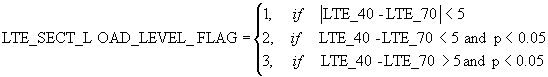(20)

where:

-          LTE_SECT_LOAD_LEVEL_FLAG = a flag indicating if section LTE depends on     FWD load level

= 1, if load level independent

= 2, if increases with load

= 3, if decreases with FWD load

-          LTE_40 = mean LTEs for the crack/joint for nominal load levels of 40 kN.

-          LTE_70 = mean LTEs for the crack/joint for nominal load levels of 70 kN.

## Results of LTE Analysis

The research team proposes that the results of the basin-by-basin LTE calculation be stored in the LTPP database table MON_DEFL_RGD_LTE_POINT.  Summary values of calculated LTE parameter results for each joint of the GPS and SPS sections should be stored in database table MON_DEFL_RGD_LTE_JOINT.  The results are presented in terms of the mean values, minimum values, maximum values, and load dependency index.  Summary values of calculated LTE parameters for each section should be stored in database table MON_DEFL_RGD_LTE_SECT.